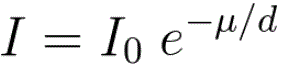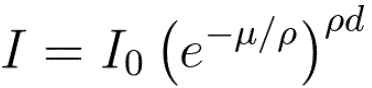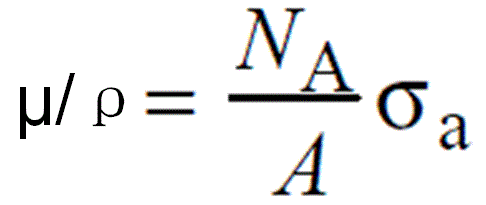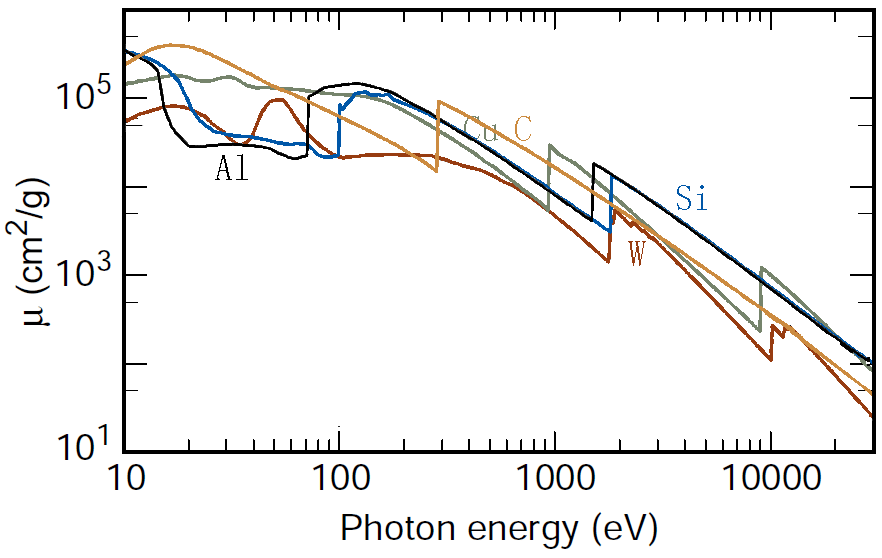Mass Absorption Coefficients of X-Rays
- Practical Electron Microscopy and Database -
- An Online Book -
Microanalysis | EM Book                                                                                   https://www.globalsino.com/EM/

=================================================================================

The intensity of the transmitted X-ray is a function of the intensity of a generated X-ray (I0), mass absorption coefficient μ/ρ (cm2/g), material density ρ (g/cm3), and thickness (cm):-------------------------------------- [1313a]

As an example, page1730 shows X-ray absorption and intensity reduction induced by carbon contamination in EMs.

Equation 1313a can be re-written as,-------------------------------------- [1313b]

Assuming all X-rays, at different wavelengths, penetrating the same materials through the same thickness, e.g. X-rays collected by EDS detector in TEM or SEM systems, then the collected X-ray intensities decreases experientially depending on the corresponding mass absorption coefficient as indicated by Equation 1313b and Figure 1313a.

Figure 1313a. .

Table 1313. Mass absorption coefficients (μ/ρ, cm2/g) of several elements.

 Co Cu Fe Mn Ni 8.04 keV (Cu Kα) 329 52 306 272 49

The mass absorption coefficient (e.g. Figure 1313b) can be given by,-------------------------------------- [1313c]
where,
NA -- Avogadro’s number,
A -- The atomic weight,
σa -- The total atomic absorption cross section (cm2/atom).Figure 1313b. Mass absorption coefficients of Al (aluminum), Cu (copper), C (carbon), W (tungsten), and Si (silicon).

The corresponding mass absorption coefficient of a compound can be calculated by the equation,
μCompound = W1μ1 + W2μ2 ... + Wnμn -------------------------------------- [1313d]
where,
Wn -- The atomic weight fractions

Note that the mass absorption coefficient for a mixture can also be calculated in the same way as shown in Equation 1313c.

=================================================================================